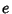## Laplace Limit

The value(Sloane's A033259) for which Laplace's formula for solving Kepler's Equation begins diverging. The constant is defined as the valueat which the functionequals. The Continued Fraction ofis given by [0, 1, 1, 1, 27, 1, 1, 1, 8, 2, 154, ...] (Sloane's A033260). The positions of the first occurrences ofin the Continued Fraction ofare 2, 10, 35, 13, 15, 32, 101, 9, ... (Sloane's A033261). The incrementally largest terms in the Continued Fraction are 1, 27, 154, 1601, 2135, ... (Sloane's A033262), which occur at positions 2, 5, 11, 19, 1801, ... (Sloane's A033263).

References

Finch, S. Favorite Mathematical Constants.'' http://www.mathsoft.com/asolve/constant/lpc/lpc.html

Plouffe, S. Laplace Limit Constant.'' http://www.lacim.uqam.ca/piDATA/laplace.txt.

Sloane, N. J. A. Sequences A033259, A033260, A033261, A033262, and A033263 in An On-Line Version of the Encyclopedia of Integer Sequences.'' http://www.research.att.com/~njas/sequences/eisonline.html.